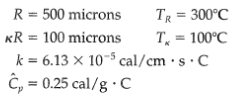### Create an Account

Home / Questions / Transpiration cooling a Calculate the temperature distribution between the two shells of E...

# Transpiration cooling a Calculate the temperature distribution between the two shells of Example 11 4 4 for radial mass flow rates of zero and

Transpiration cooling
(a) Calculate the temperature distribution between the two shells of Example 11.4-4 for radial mass flow rates of zero and 10 -5 g/s for the following conditions:
(b) Compare the rates of heat conduction to the surface at KR in the presence and absence of convection.Jul 25 2020 View more View LessSubscribe To Get Solution